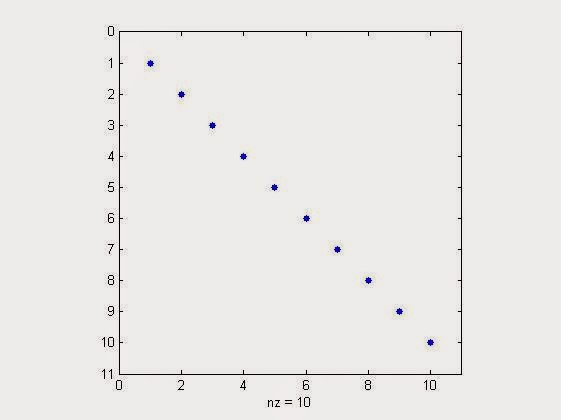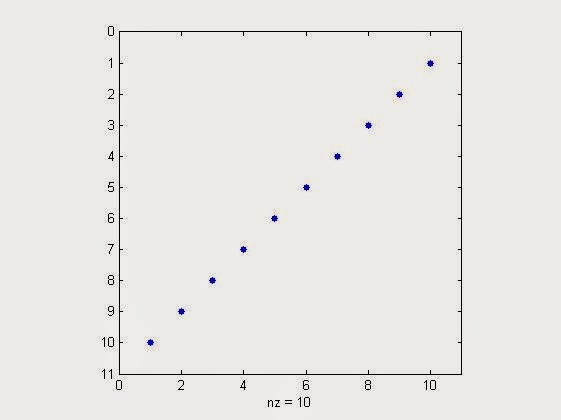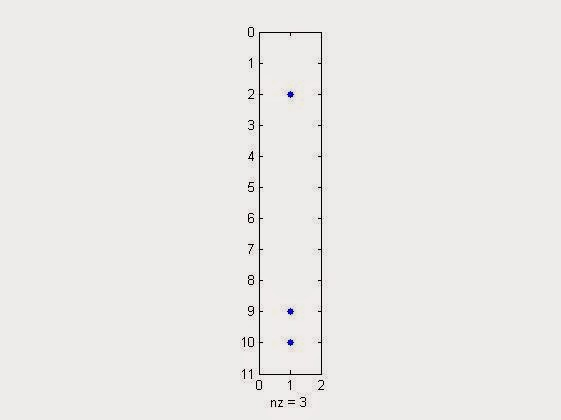# How to Visualize Sparsity of a Matrix or a Vector in MATLAB?

So, what is sparse signal/matrix/vector? Their generation & use in Matlab refer the link of our previous article, Creating & Using Sparse Matrix & Vectors in MATLAB - Concepts & Examples.

Now its time to visualize the sparsity of generated or received signal/vector/matrix. But why there is a need of visualization?
The actual need arises when we want to see where the energy is concentrated  in the received or generated vector/matrix. If the matrix/vector is sparse then there are very few percentage of non-zero elements, thus energy will be more concentrated to those elements.

The command we will use for this purpose is spy( ). Remember this command has got its place at Digital iVision Labs, earlier also in the article : Unusual Commands of MATLAB. It will give output as, when used without any parameters or function.Spy Command without any parameter
Now coming towards the topic of visualizing sparsity.
Suppose 'x'  is a sparse matrix, having 60x60 dimensionality. Then  we need to use, the command as "spy(x)" to visualize the sparsity in matlab.
Following matlab's own example of "bucky",
NOTE:  bucky is Connectivity graph of the Buckminster Fuller geodesic dome. Bucky is the 60-by-60 sparse adjacency matrix of the

Commands & Output
>> x=bucky; %generating a 60x60 sparse matrix
>> spy(x) % to visualise the sparsity.

It will pop up a graph plot as,Bucky Sparsity Visualization
Now playing around with some test more cases of sparsity visualization,
>> spy(speye(10)) %sparsity visualization of 10x10 identity matrix
It will popup the graph plot as,sparsity visualization of 10x10 identity matrix in matlab
>> spy(flipud(speye(10))) %sparsity visualization of 10x10 identity matrix flipped up side down
It will popup the graph plot as,%sparsity visualization of 10x10 identity matrix flipped up side down in matlab

Similarly testing it with a colunm vector,
>> spy(sprand(10,1,0.3)) % generate sparse visualisation plot for a sparse 10x1 column vector with a sparsity of 30%
Result will be,sparse visualization plot for a sparse 10x1 column vector with a sparsity of 30% in matlab
Many things waiting for you at Digital iVision Labs!

Read More Swapping Rows & Columns of Matrix in MATLAB